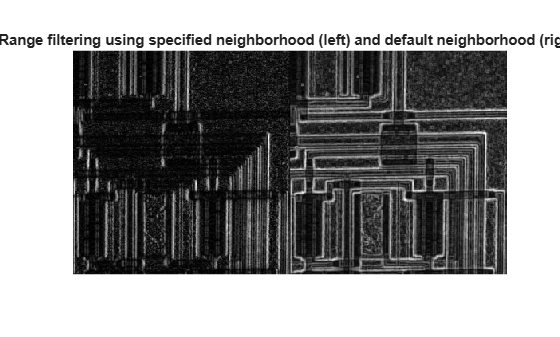# rangefilt

Local range of image

## Syntax

``J = rangefilt(I)``
``J = rangefilt(I,nhood)``

## Description

example

````J = rangefilt(I)` returns the array `J`, where each output pixel contains the range value (maximum value − minimum value) of the 3-by-3 neighborhood around the corresponding pixel in the input image `I`.```

example

````J = rangefilt(I,nhood)` returns the local range of image `I` using the specified neighborhood, `nhood`.```

## Examples

collapse all

Read an image into the workspace.

`I = imread('liftingbody.png'); `

Filter the image. The `rangefilt` function returns an array where each output pixel contains the range value (maximum value - minimum value) of the 3-by-3 neighborhood around the corresponding pixel in the input image.

```J = rangefilt(I); ```

Display the original image and the filtered image side-by-side.

`imshowpair(I,J,'montage')`This example shows how to detect regions of texture in an image using the texture filter functions

Read an image into the workspace and display it. In the figure, the background is smooth—there is very little variation in the gray-level values. In the foreground, the surface contours of the coins exhibit more texture. In this image, foreground pixels have more variability and thus higher range values.

```I = imread('eight.tif'); imshow(I)```Filter the image with the `rangefilt` function and display the results. Range filtering makes the edges and contours of the coins visible.

```K = rangefilt(I); figure imshow(K)```Read an image into the workspace, and display it.

```I = imread('circuit.tif'); imshow(I);```Define a neighborhood. In this example, the neighborhood returns a large value when there is a large difference between pixel values to the left and right of an input pixel. The filtering does not consider pixels above and blow the input pixel. Thus, this neighborhood emphasizes vertical edges.

`nhood = [1 1 1];`

Perform the range filtering operation using this neighborhood. For comparison, also perform range filtering using the default 3-by-3 neighborhood. Compare the results.

```J = rangefilt(I,nhood); K = rangefilt(I); figure imshowpair(J,K,'montage'); title('Range filtering using specified neighborhood (left) and default neighborhood (right)');```The result using the specified neighborhood emphasizes vertical edges, as expected. In comparison, the default filter is not sensitive to edge directionality.

## Input Arguments

collapse all

Image to be filtered, specified as a numeric array of any dimension.

Data Types: `double` | `uint8` | `uint16` | `uint32` | `logical`

Neighborhood, specified as a logical or numeric array containing zeros and ones. The size of `nhood` must be odd in each dimension. `rangefilt` determines the center element of the neighborhood by `floor((size(NHOOD) + 1)/2)`.

To specify neighborhoods of other shapes, such as a disk, use the `strel` function to create a structuring element object of the desired shape. Then, extract the neighborhood from the structuring element object’s `neighborhood` property.

Data Types: `single` | `double` | `int8` | `int16` | `int32` | `int64` | `uint8` | `uint16` | `uint32` | `uint64` | `logical`

## Output Arguments

collapse all

Filtered image, returned as a numeric array, the same size and class as the input image `I`, except for signed integer data types. The output class for signed data types is the corresponding unsigned integer data type. For example, if the class of `I` is `int8`, then the class of `J` is `uint8`.

## Algorithms

`rangefilt` uses the morphological functions `imdilate` and `imerode` to determine the maximum and minimum values in the specified neighborhood. Consequently, `rangefilt` uses the padding behavior of these morphological functions.

## Version History

Introduced before R2006a